# 1140 Exam1Review v2 .pdf

### File information

Original filename: 1140_Exam1Review_v2.pdf

This PDF 1.5 document has been generated by LaTeX with hyperref package / pdfTeX-1.40.14, and has been sent on pdf-archive.com on 22/09/2017 at 14:32, from IP address 216.155.x.x. The current document download page has been viewed 261 times.
File size: 406 KB (4 pages).
Privacy: public file

1140_Exam1Review_v2.pdf (PDF, 406 KB)

### Document preview

MAC 1140 Exam 1 Review

Fall 2016

This review, produced by the CLAS Teaching Center, contains a collection of questions which are
representative of the type you may encounter on the exam. Other resources made available by the
Teaching Center include:
• Walk-In tutoring at Broward Hall
• Private-Appointment, one-on-one tutoring at Broward Hall
• Walk-In tutoring in LIT 215
• Supplemental Instruction
• Video resources for Math and Science classes at UF
• Written exam reviews and copies of previous exams

The teaching center is located in the basement of Broward Hall:

https://teachingcenter.ufl.edu/

MAC 1140 Exam 1 Review
1. Sketch the following subsets of the real numbers on a number line
(a) [−4, 12)
(b) (−∞, 0]
(c) x − 3 ≤ 5
(d) x is no larger than 9.
2. Sketch the following subsets of the real numbers on a number line
(a) |x| &lt; 2
(b) |x| ≥ 2
(c) |x − 1| &gt; 3
(d) The distance from x to 1 is at least 2.

18z 5 x7
(a) √
2zx3

5
(b) 64x10 − 32x5

√2 ·
12 + 12
2
(c)
2
4. Write each function piecewise without absolute value bars.
(a) e(x) = |x|
(b) f (x) = |2x + 1|
|10x + 8|
5x + 4
1
(d) h(x) =
|x|
(c) g(x) =

5. Expand each of the following expressions.
(a) (x + 1)2
(b) (x − 2)(x + 2)
(c) (2x − 1)3
(d) (x + y + 1)(x + y − 1)

CLAS Teaching Center

2

MAC 1140 Exam 1 Review
6. Determine the requested coefficient in the expanded form of each expression below.
(a) Coefficient of x2 in (2x − 4)(1 − x)
(b) Coefficient of x in (2x + 1)2
(c) Coefficient of x3 in (2x + 3)3
(d) Coefficient of xy in (x + y − 1)(2x − 3y + 2)
7. Factor each of the following polynomials.
(a) 8y 2 (x + 3) − 2(x + 3)
(b) x4 − 4
(c) x2 + x − 6
(d) 3x2 − 6x + 3
(e) 2x2 + 5x − 3
(f) x3 − 27
(g) x3 + 3x2 − 6x − 18
8. Simplify and find the domain of each rational function.
1
(a) f (x) =
1−x
x+1
(b) g(x) = 2
x +x−6
9. Simplify each expression, leaving positive exponents only.
(a) 3x−4/3 + 2x−1/3
(b) −x−1 (1 + x2 )−2/3 − 2x−3 (1 + x2 )1/3
(c) x2 (1 − 2x)−3/2 + (1 − 2x)−1/2
(d)

−2(x2 − 3)−3 (2x)(x + 1)3 − 3(x + 1)2 (x2 − 3)−2
[(x + 1)3 ]2

(e)

(6x + 1)3 (27x2 + 2) − (9x3 + 2x)(3)(6x + 1)2 (6)
[(6x + 1)3 ]2

10. Find the domain of each of the following functions.
x−3
(a) g(x) = 2
x − 5x + 10

(b) h(x) = 2x + 7

CLAS Teaching Center

3

MAC 1140 Exam 1 Review
11. Solve the following equations for the indicated variable.
x+2
(a) y =
for x.
x−1
(b) 4x2 − 1 = 7 for x

(c) 3 − 2t = t for t
(d) |x − 2| = 1 for x

(e) x + 31 − 9x = 5 for x
10x + 3
1
(f)
= for x
5x + 6
2
(g) (x2 − x − 22)3/2 = 27 for x
12. Solve the following inequalities.
(a) −2x + 1 ≥ 0
(b) |x − 5| + 15 &gt; 5

CLAS Teaching Center

4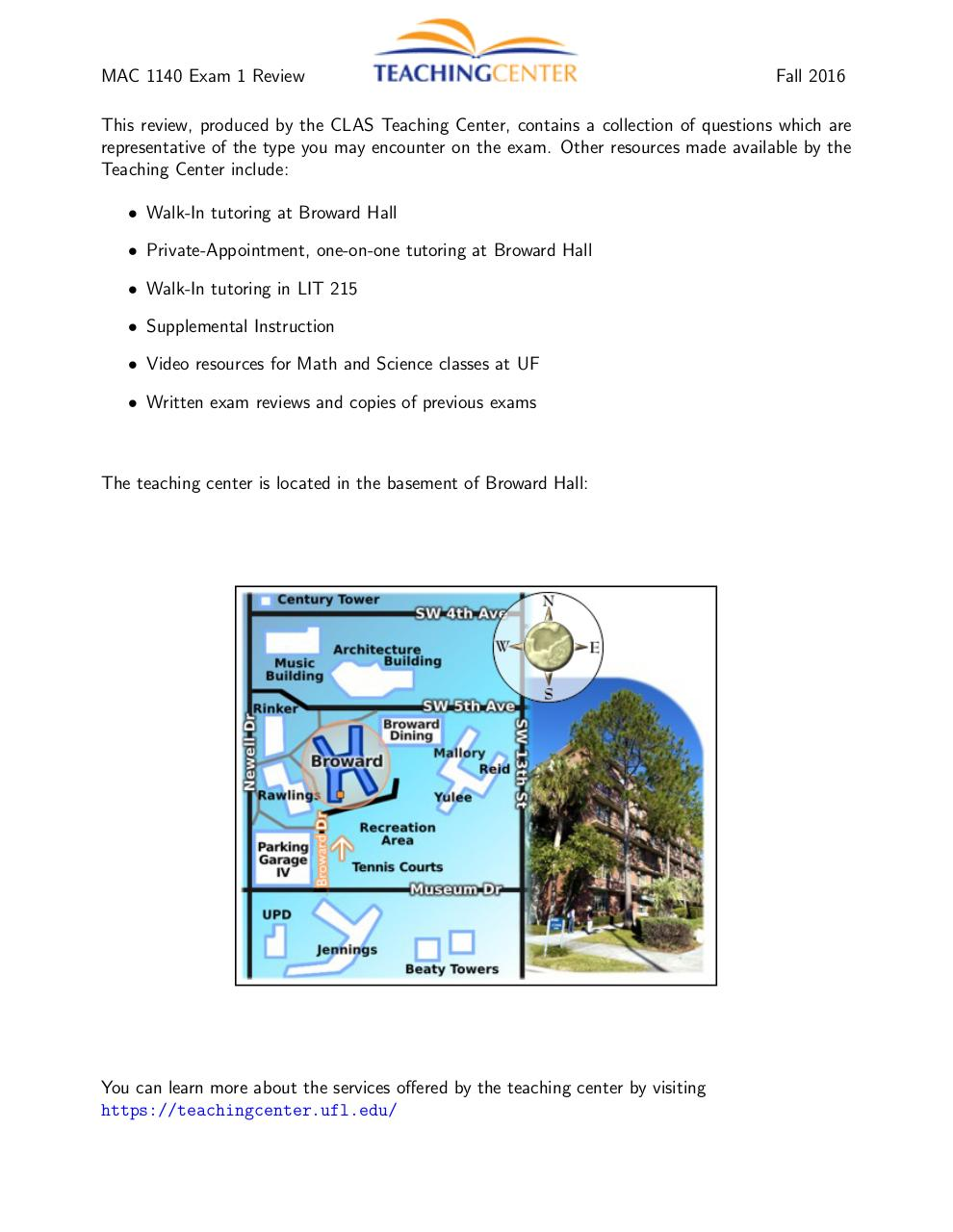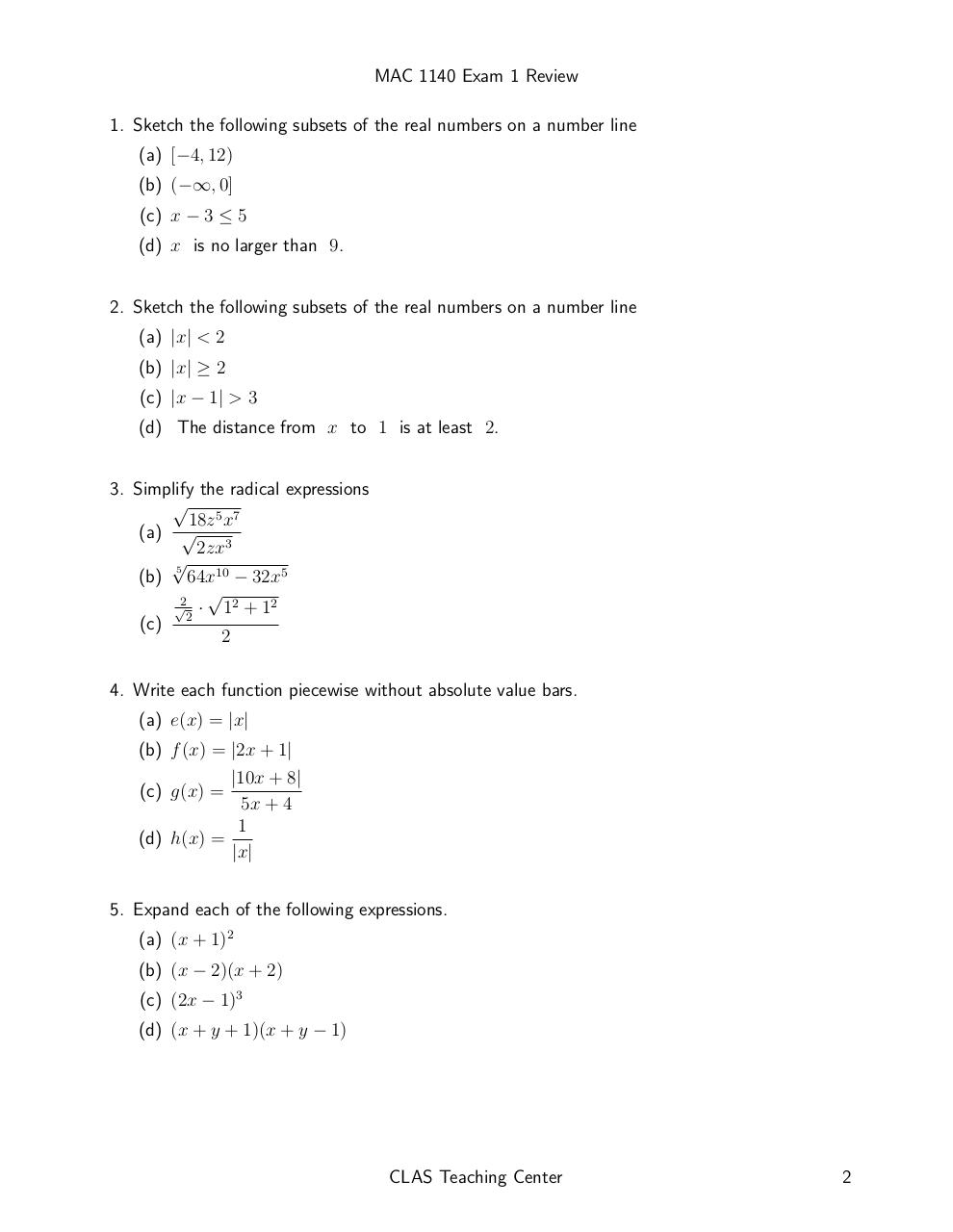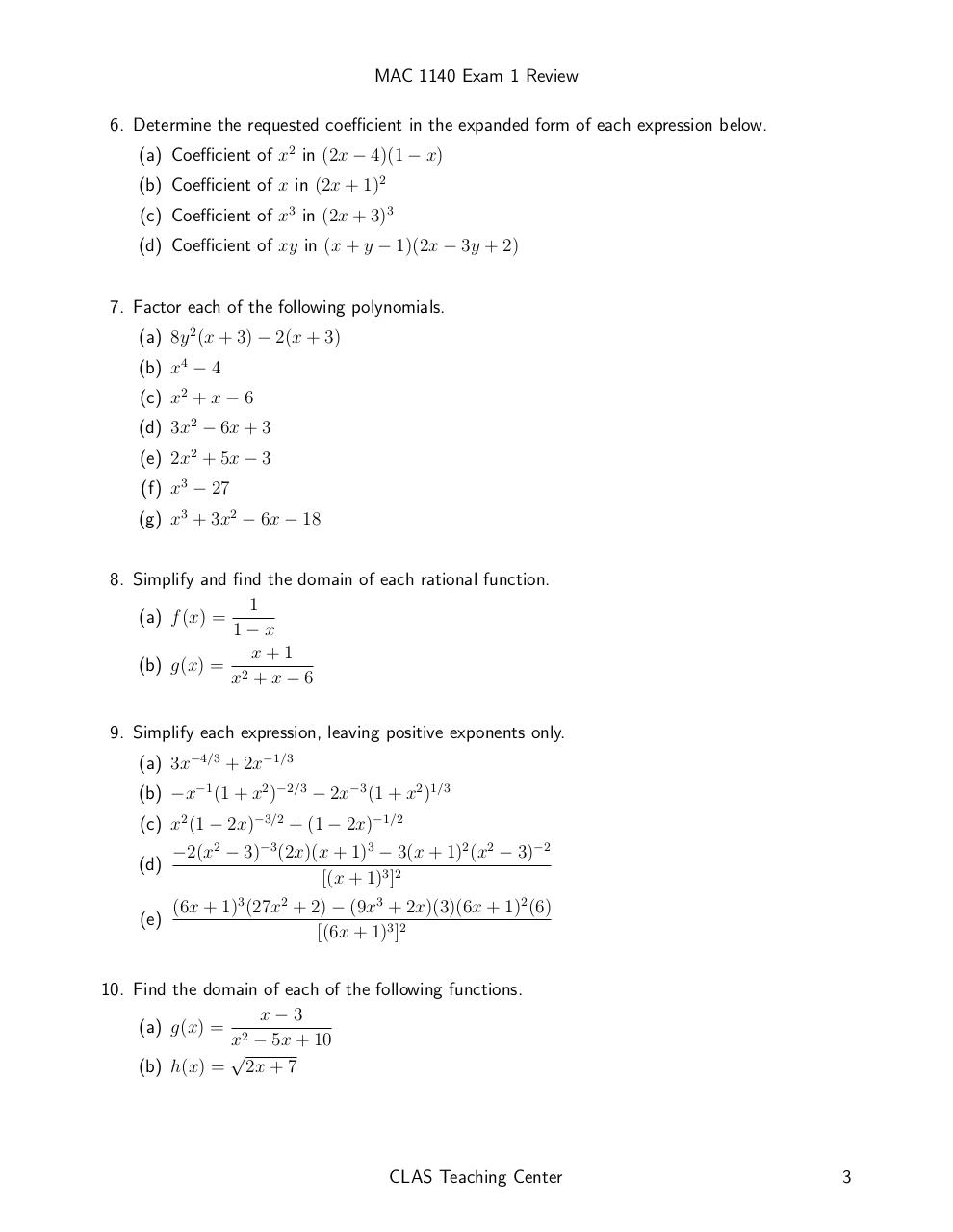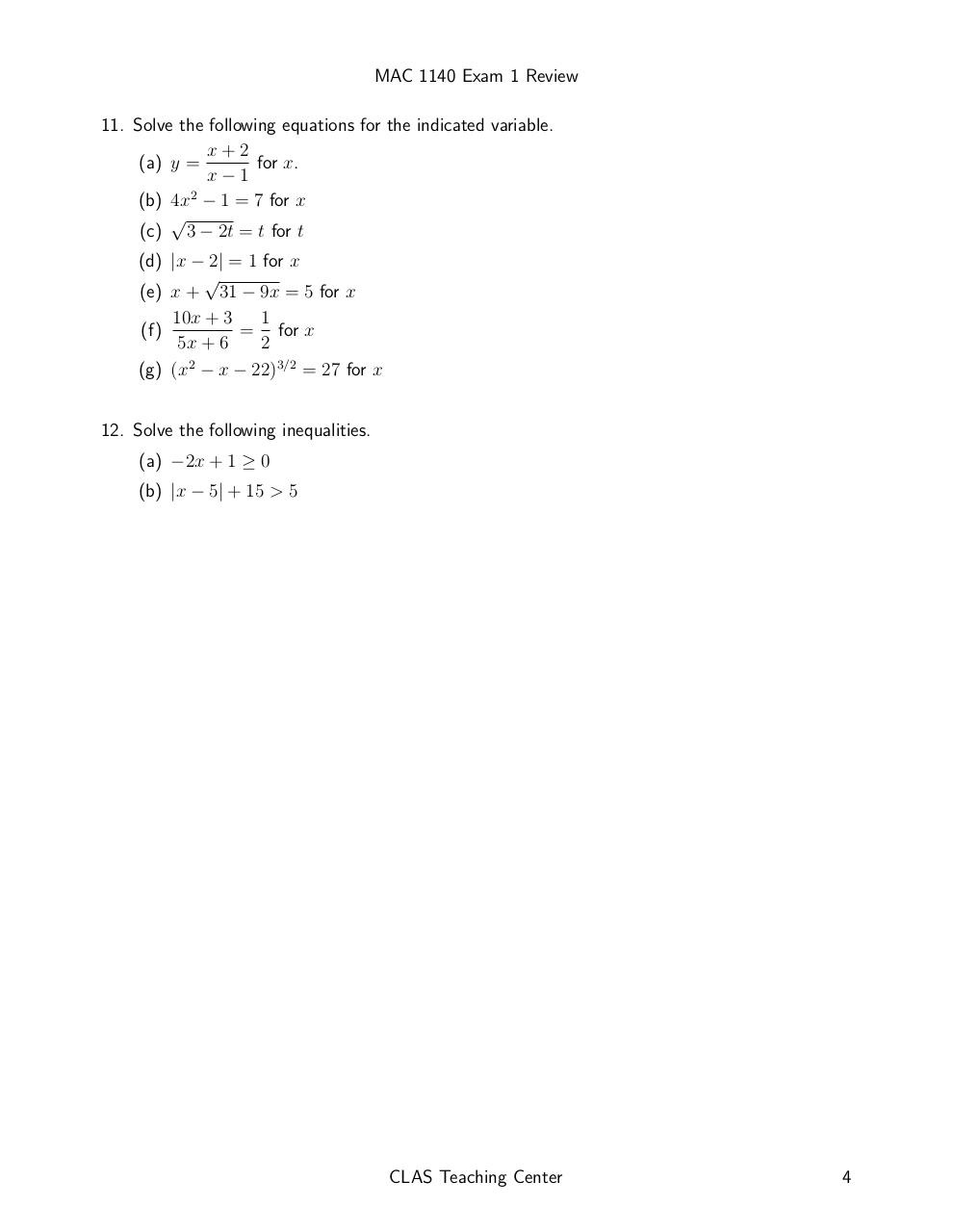#### HTML Code

Copy the following HTML code to share your document on a Website or Blog

#### QR Code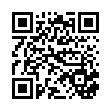### Related keywords